# Adding and Subtracting Scientific Notation Lesson Plan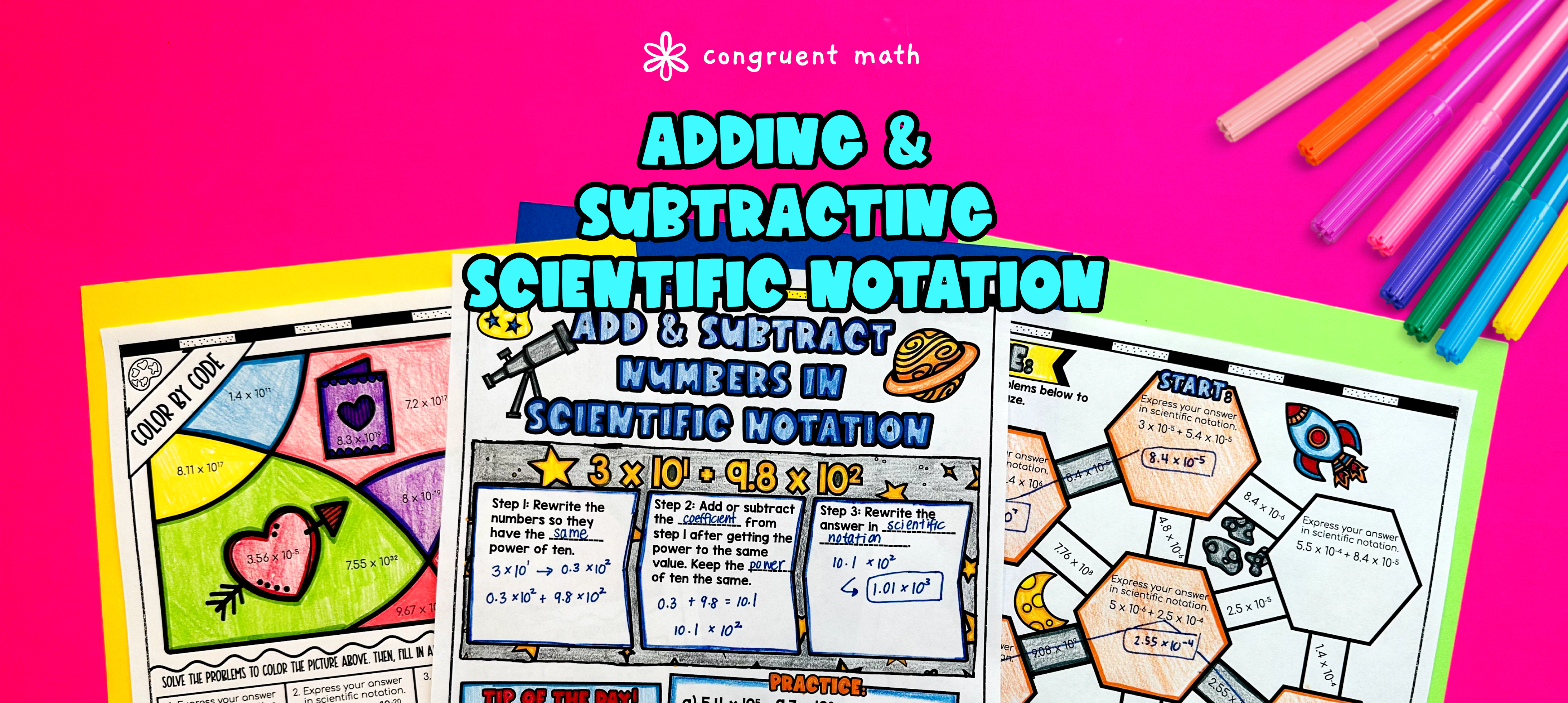Pin This

## Overview

Ever wondered how to teach adding and subtracting numbers in scientific notation in an engaging way to your eighth-grade students?

In this lesson plan, students will learn about scientific notations and their real-life applications. Through artistic, interactive guided notes, check for understanding, a doodle & color by number activity, and a maze worksheet, students will gain a comprehensive understanding of adding and subtracting numbers in scientific notation.

The lesson culminates with a real-life example that explores how adding and subtracting numbers in scientific notation is used in practical situations.

## Get the Lesson Materials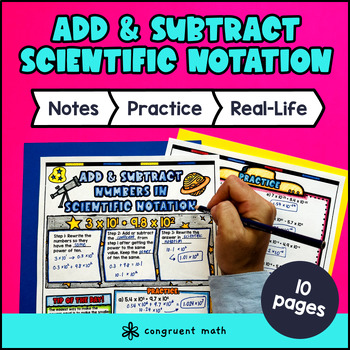\$4.25

## Learning Objectives

After this lesson, students will be able to:

• Understand the concept of scientific notation and its purpose in representing large and small numbers

• Add and subtract numbers expressed in scientific notation

• Recognize and explain the importance of adding and subtracting numbers in scientific notation in various fields such as science, engineering, and economics.

## Prerequisites

Before this lesson, students should be familiar with:

• Basic operations of addition and subtraction of integers

• Understanding of place value and exponents

## Key Vocabulary

• Scientific Notation

• Exponent

• Power of 10

• Coefficient

• Standard Form

## Procedure

### Introduction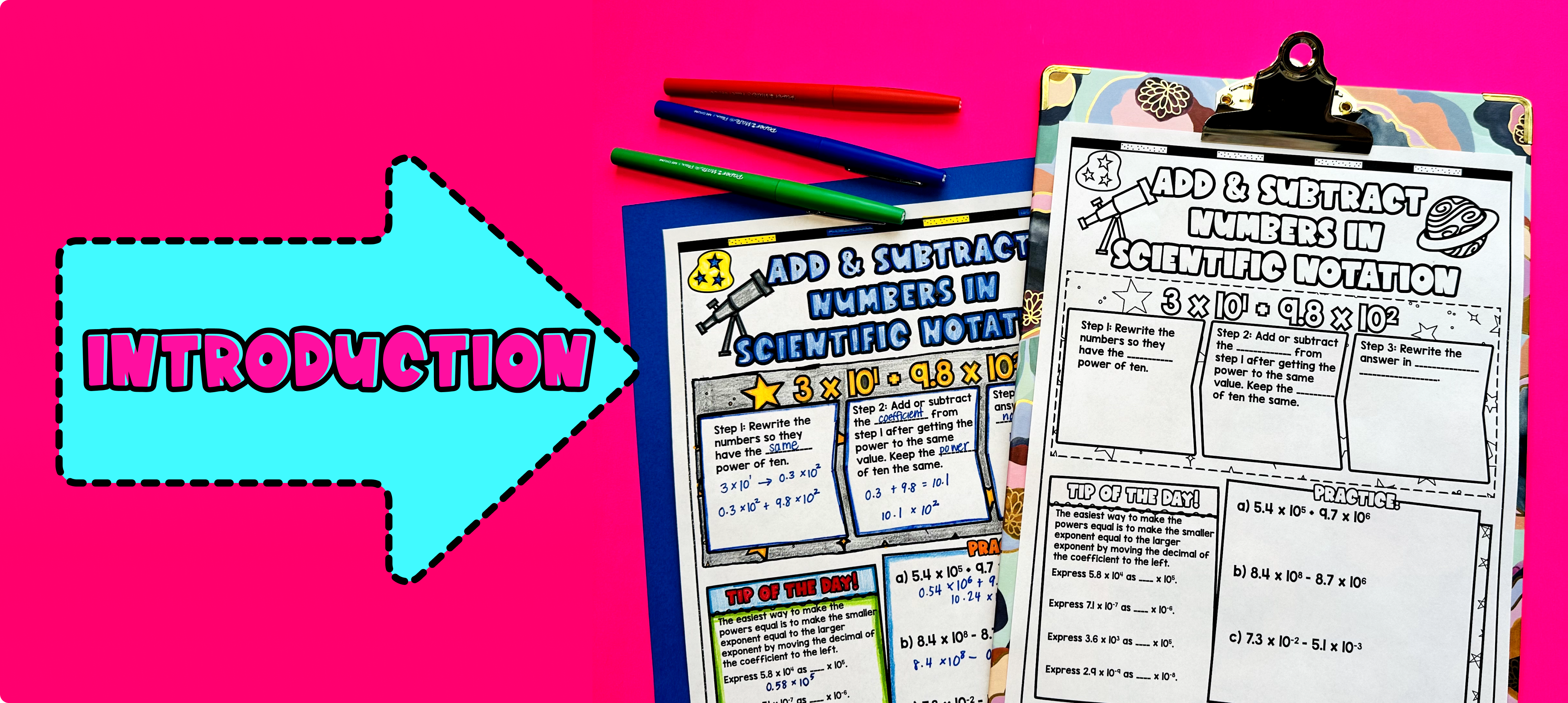Pin This

As a hook, ask students why adding and subtracting numbers in scientific notation is important in real-life applications. Refer to the last page of the guided notes as well as the FAQs below for ideas.

Use the first page of the guided notes to introduce adding and subtracting numbers expressed in scientific notation. Walk through the key points of the topic, including how to add and subtract the numbers and how to rewrite the numbers in scientific notation form. Refer to the FAQ below for a walk through on this, as well as ideas on how to respond to common student questions.

Based on student responses, reteach concepts that students need extra help with. If your class has a wide range of proficiency levels, you can pull out students for reteaching, and have more advanced students begin work on the practice exercises.

### Practice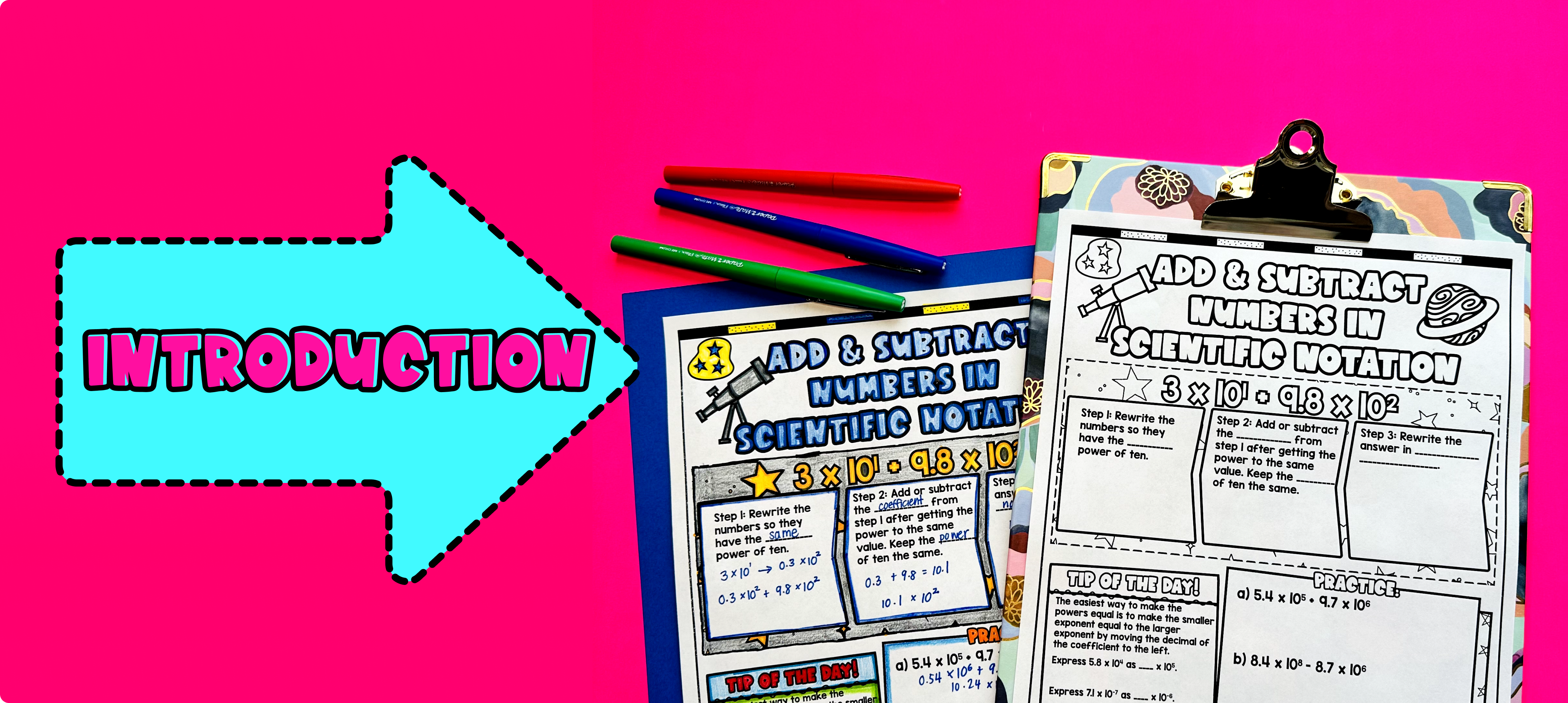Pin This

Have students practice adding and subtracting numbers in scientific notation using the practice worksheet on the second page of guided notes. Walk around to answer student questions.

Fast finishers can dive into the maze activity (page 3 of guided notes) and color by number (page 4 of guided notes) for extra practice. You can assign it as homework for the remainder of the class.

### Real-Life Application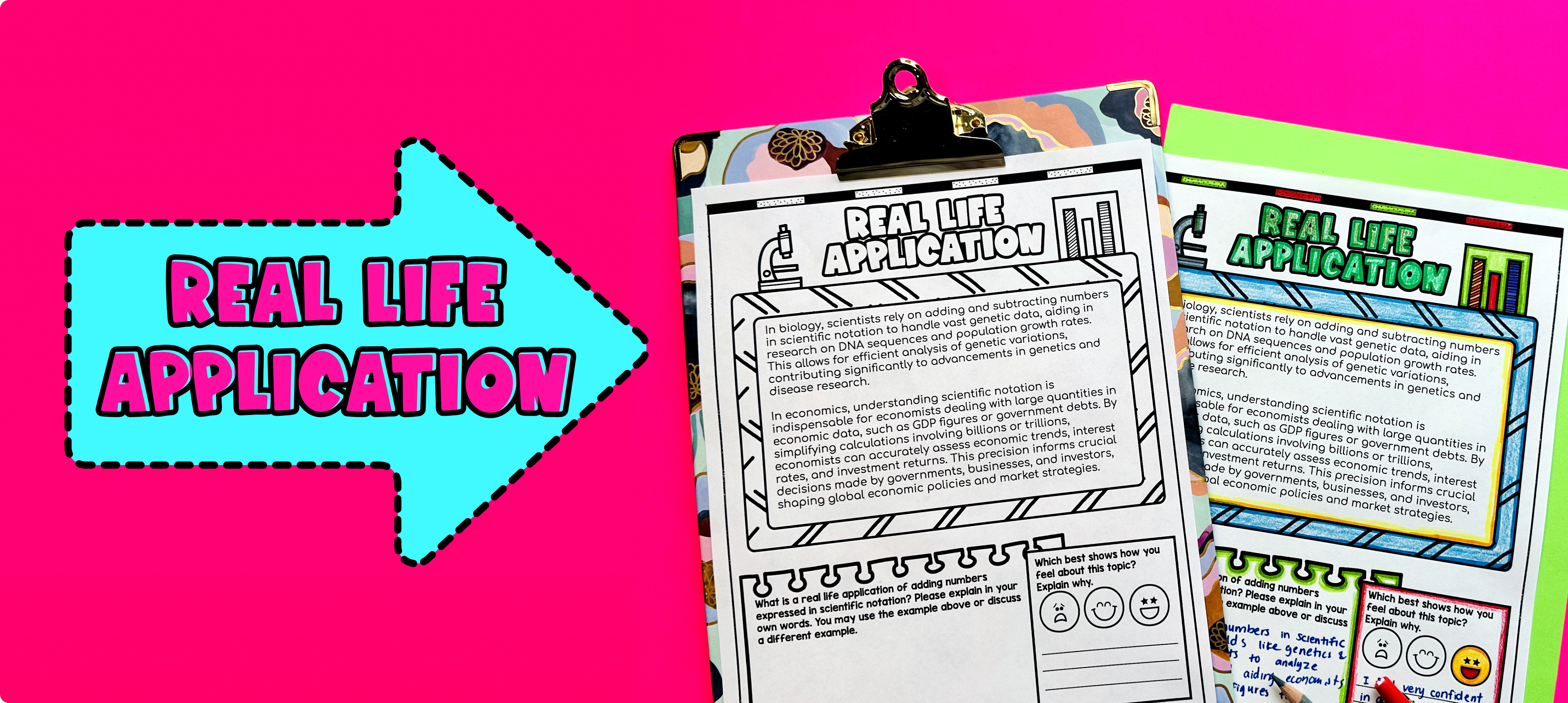Pin This

Bring the class back together, and introduce the concept of real-life uses of adding and subtracting numbers in scientific notation. Use the last page of the guided notes for students to read a detailed example of adding and subtracting numbers in scientific notation form. Explain that scientific notation is commonly used in various fields of science, such as astronomy, physics, and chemistry, where extremely large or small numbers are encountered.

To illustrate the real-life application, provide the students with examples of situations where scientific notation is used generally. Some examples could include:

1. Astronomical Distances: In astronomy, scientists often work with incredibly large distances, such as the distance between stars or galaxies. Expressing these distances in standard form would result in numbers with many zeros. For example, the distance from the Sun to the nearest star, Proxima Centauri, is approximately 4.22 × 10^13 kilometers.

2. Molecules and Atoms: In the field of chemistry, scientists often deal with extremely small numbers, such as the number of atoms or molecules in a substance. For instance, a single drop of water contains approximately 3.34 × 10^22 molecules.

3. Earthquakes: When measuring the intensity of earthquakes, scientists use the Richter scale, which represents the magnitude of seismic waves caused by the earthquake. The Richter scale uses scientific notation to express the energy released during an earthquake. For example, a magnitude 7 earthquake releases about 2.0 × 10^15 joules of energy.

Ask the students to brainstorm other examples they can think of where scientific notation is useful in real life. Encourage discussion and allow them to share their ideas with the class.

Refer to the FAQ section for more ideas on how to incorporate real-life applications into this lesson.

## Extensions

If you’re looking for digital practice for adding and subtracting numbers in scientific notation, try my Pixel Art activities in Google Sheets. Every answer is automatically checked, and correct answers unlock parts of a mystery picture. It’s incredibly fun, and a powerful tool for differentiation.

Here are some activities to explore: